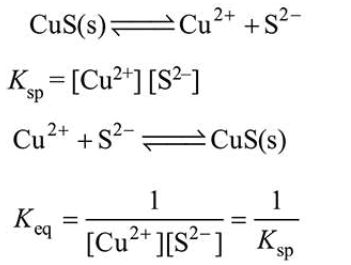# On heating compound (A) gives a gas (B) which is a constituent of air.

Question:

On heating compound (A) gives a gas (B) which is a constituent of air. This gas when treated with $\mathrm{H}_{2}$ in the presence of a catalyst gives another gas (C) which is basic in nature. (A) should not be :

1. $\mathrm{NaN}_{3}$

2.  $\left(\mathrm{NH}_{4}\right)_{2} \mathrm{Cr}_{2} \mathrm{O}_{7}$

3. $\mathrm{Pb}\left(\mathrm{NO}_{3}\right)_{2}$

4. $\mathrm{NH}_{4} \mathrm{NO}_{2}$

Correct Option: , 3

Solution:

$\mathrm{Cu}^{2+}$ ions get precipitated every quickely due to low $K_{\mathrm{sp}}$ value even at very low concentration of $\mathrm{S}^{2-}$ ion.Due to high value of $K_{\text {eq }}$, CuS precipitated easily.